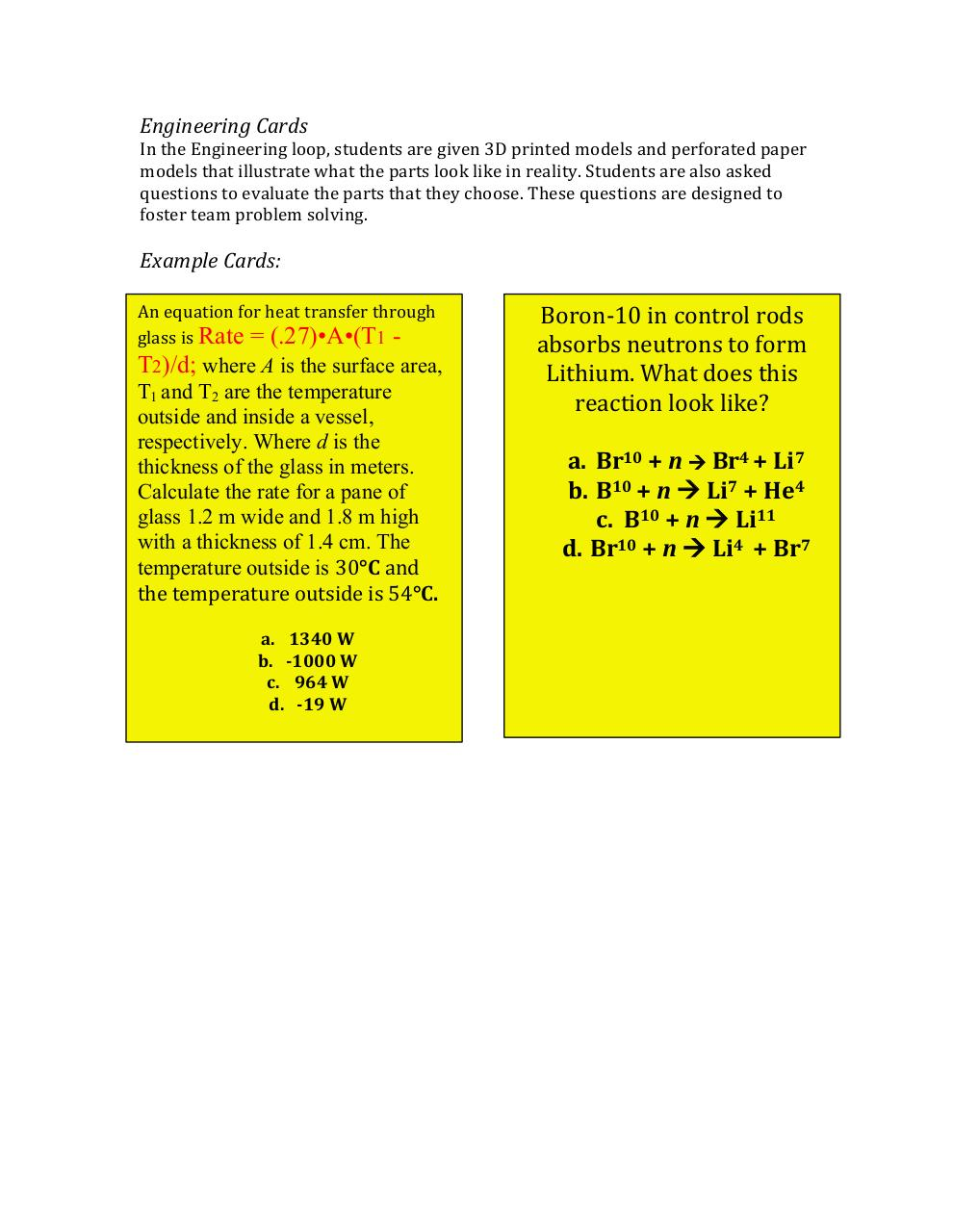# Power Generation Design Strategy Board Game.pdfPage 1 2 3 4 5 6 7 8

#### Text preview

Engineering Cards
In the Engineering loop, students are given 3D printed models and perforated paper
models that illustrate what the parts look like in reality. Students are also asked
questions to evaluate the parts that they choose. These questions are designed to
foster team problem solving.

Example Cards:
An equation for heat transfer through
glass is Rate = (.27)•A•(T1 -

T2)/d; where A is the surface area,
T1 and T2 are the temperature
outside and inside a vessel,
respectively. Where d is the
thickness of the glass in meters.
Calculate the rate for a pane of
glass 1.2 m wide and 1.8 m high
with a thickness of 1.4 cm. The
temperature outside is 30°C and
the temperature outside is 54°C.
a. 1340 W
b. -1000 W
c. 964 W
d. -19 W

Boron-10 in control rods
absorbs neutrons to form
Lithium. What does this
reaction look like?
a. Br10 + n  Br4 + Li7
b. B10 + n  Li7 + He4
c. B10 + n  Li11
d. Br10 + n  Li4 + Br7